# Varactor Diode

Varactor Diode is a reverse-biased p-n junction diode, whose capacitance can be varied electrically. As a result these diodes are also referred to as varicaps, tuning diodes, voltage variable capacitor diodes, parametric diodes, and variable capacitor diodes.

It is well known that the operation of the p-n junction depends on the bias applied which can be either forward or reverse in characteristic. It is also observed that the span of the depletion region in the p-n junction decreases as the voltage increases in case of forward bias.

On the other hand, the width of the depletion region is seen to increase with an increase in the applied voltage for the reverse bias scenario.

Under such conditions, the p-n junction can be considered to be analogous to a capacitor (Figure 1) where the p and n layers represent the two plates of the capacitor while the depletion region acts as a dielectric separating them.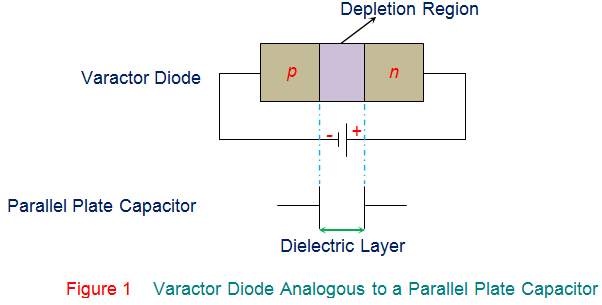Thus one can apply the formula used to compute the capacitance of a parallel plate capacitor even to the varactor diode.

Hence, the mathematical expression for the capacitance of varactor diode is given by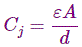Where,
Cj is the total capacitance of the junction.
ε is the permittivity of the semiconductor material.
A is the cross-sectional area of the junction.
d is the width of the depletion region.

Further, the relationship between the capacitance and the reverse bias voltage is given asWhere,
Cj is the capacitance of the varactor diode.
C is the capacitance of the varactor diode when unbiased.
K is the constant, often considered to be 1.
Vb is the barrier potential.
VR is the applied reverse voltage.
m is the material-dependent constant.

In addition, the electrical circuit equivalent of a varactor diode and its symbol is shown in Figure 2.

This indicates that the maximum operating frequency of the circuit is dependent on the series resistance (Rs) and the diode capacitance, which can be mathematically given as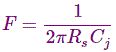In addition, the quality factor of the varactor diode is given by the equationWhere, F and f represent the cut-off frequency and the operating frequency, respectively.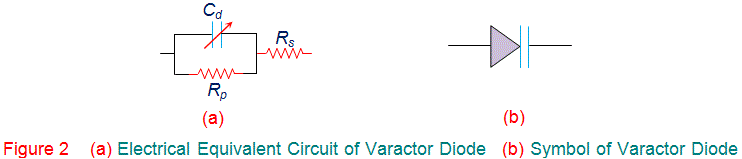As a result, one can conclude that the capacitance of the varactor diode can be varied by varying the magnitude of the reverse bias voltage as it varies the width of the depletion region, d. Also it is evident from the capacitance equation that d is inversely proportional to C. This means that the junction capacitance of the varactor diode decreases with an increase in the depletion region width caused to due to an increase in the reverse bias voltage (VR), as shown by the graph in Figure 3. Meanwhile it is important to note that although all the diodes exhibit the similar property, varactor diodes are specially manufactured to achieve the objective. In other words varactor diodes are manufactured with an intention to obtain a definite C-V curve which can be accomplished by controlling the level of doping during the process of manufacture. Depending on this, varactor diodes can be classified into two types viz., abrupt varactor diodes and hyper-abrupt varactor diodes, depending on whether the p-n junction diode is linearly or non-linearly doped (respectively).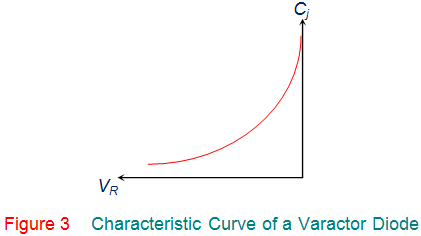These varactor diodes are advantageous as they are compact in size, economical, reliable and less prone to noise when compared to other diodes. Hence, they are used in

1. Tuning circuits to replace the old style variable capacitor tuning of FM radio
2. Small remote control circuits
3. Tank circuits of receiver or transmitter for auto-tuning as in case of TV
4. Signal modulation and demodulation.
5. Microwave frequency multipliers as a component of LC resonant circuit
6. Very low noise microwave parametric amplifiers
7. AFC circuits